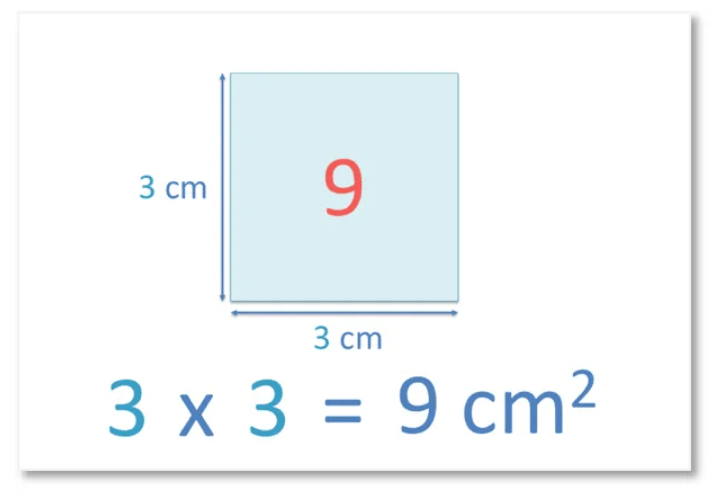# The 11 Times Table

The 11 Times TableThe 11 times table is:

• 1 × 11 = 11
• 2 × 11 = 22.
• 3 × 11 = 33
• 4 × 11 = 44
• 5 × 11 = 55
• 6 × 11 = 66
• 7 × 11 = 77
• 8 × 11 = 88
• 9 × 11 = 99
• 10 × 11 = 110
• 11 × 11 = 121
• 12 × 11 = 132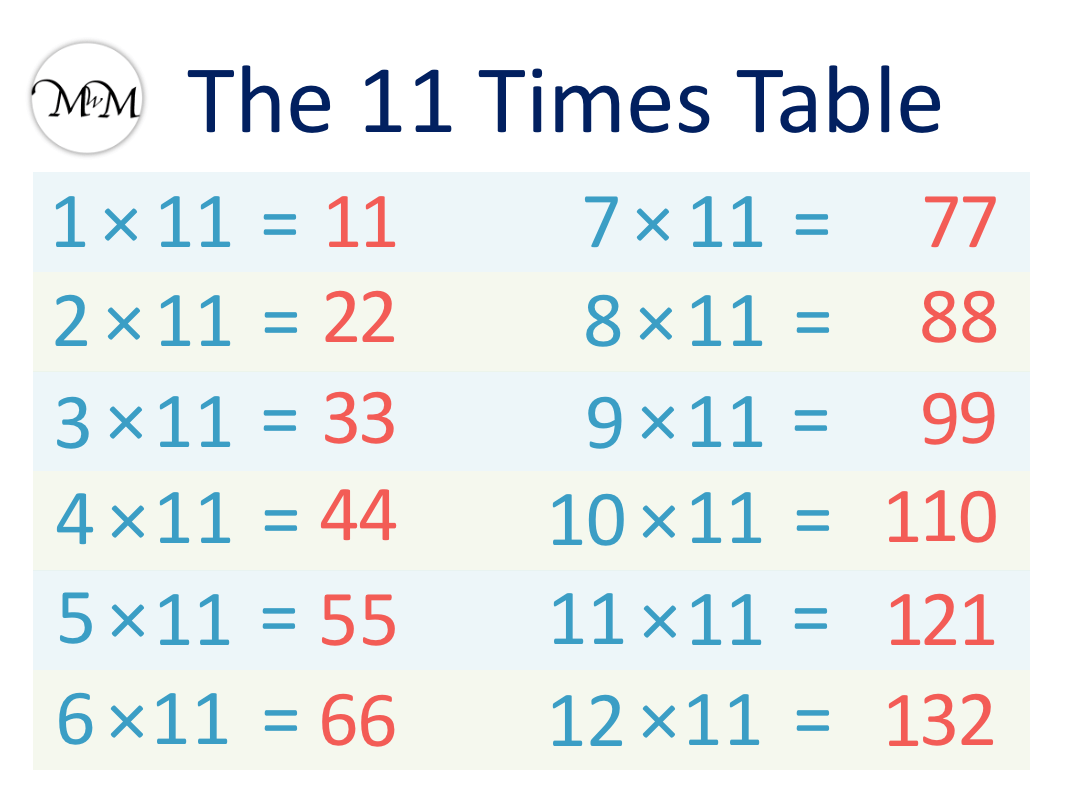• The 11 times table is made by counting up in elevens.
• To multiply a number from 1 to 9 by 11, simply repeat the digit.
• We can remember 11 × 11 = 121 and 12 × 11 = 132 because the outer digits of each answer add up to make the middle digit.

To multiply a single digit number by 11, simply repeat the digit.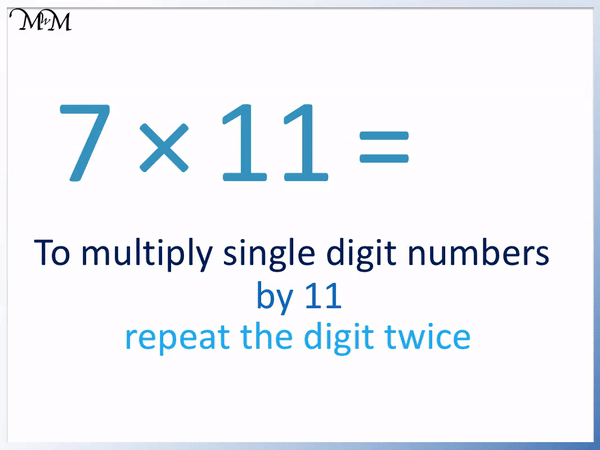• Here is 7 × 11.
• To multiply by 11, we simply repeat the digit.
• We repeat the digit of 7 to get 77.
• 7 × 11 = 77.#### 11 Times Table Flashcards

Click on the 11 times table flashcards below to memorise the 11 times table.

×
×

=

# The 11 Times Table

Here is the complete 11 times table chart.## 11 Times Table Trick

The times table trick for multiplying a single digit by 11 is to repeat the digit.

For example, to multiply 5 by 11, repeat the digit of 5. Therefore 5 multiplied by 11 is 55.

Here is the 11 times table chart showing this times table trick.

We repeat each digit from 1 to 9 when multiplying them by 11.Here are some examples of using this times table trick to multiply single digit numbers by 11.

The rule is to multiply single digit numbers by 11, repeat the digit.

Here is the example of 2 × 11 = 22.

We simply repeat the digit of 2 to get 22.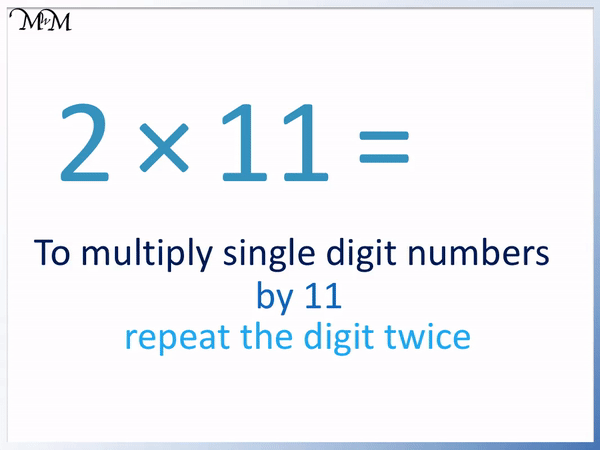In this example we multiply 5 by 11.

We repeat the digit of 5 so that 5 × 11 = 55.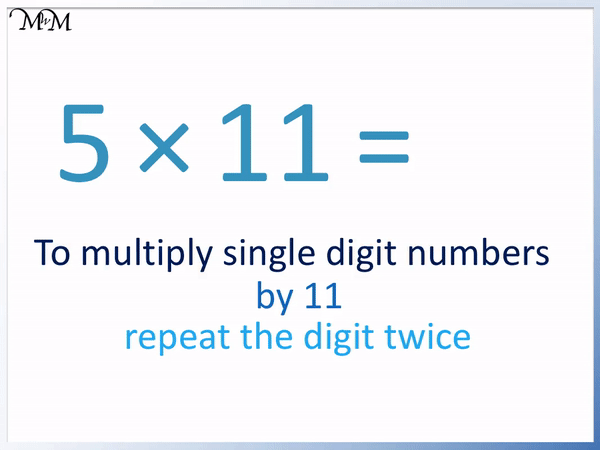In this example we multiply 7 by 11.

We repeat the digit 7 so that 7 × 11 = 77.To remember the remaining numbers in the 11 times table, we can use some further tricks.

To multiply a whole number by 10, we simply put a zero digit on the end.

So 10 × 11 = 110.

11 × 11 = 121 and 12 × 11 = 132.

We can use another trick to remember the answers to 10, 11 and 12 times 11.

All three of these answers begin with 1, end in the same digit as 10, 11 or 12 and the middle digit is the sum of the two outer digits.

For example 10 × 11 = 110.110 ends in a 0 like 10 does. 110 starts with a 1 and the middle digit is the sum of these outer digits, 1 and 0.

11 × 11 = 121.121 ends in a 1 like 11 does. 121 starts with a 1 and the middle digit is the sum of these outer digits, 1 + 1.

12 × 11 = 132.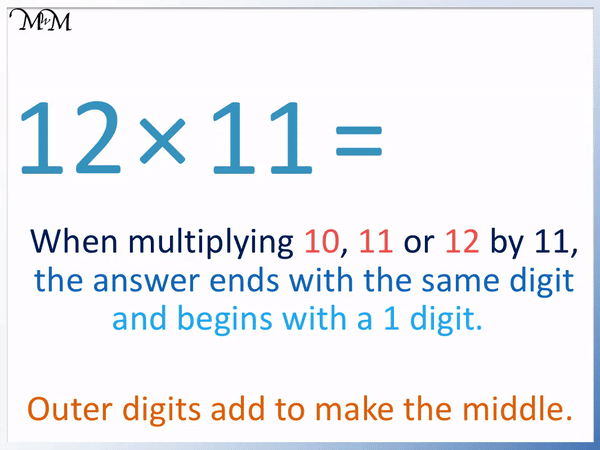132 ends in a 2 like 2 does. 132 starts with a 2 and the middle digit is the sum of these outer digits, 1 + 2.Now try our lesson What are Square Numbers? where we learn how to find square numbers.